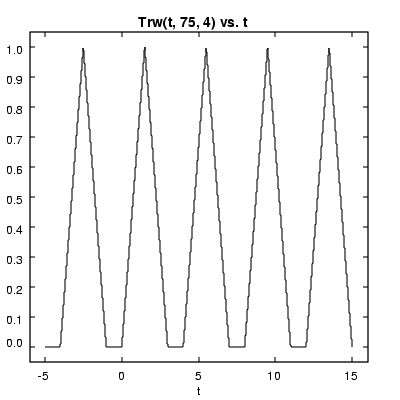ODE Toolkit recognizes the following pre-defined functions:

Trigonometric functions

 sin(x) sine of x radians cos(x) cosine of x radians tan(x) tangent of x radians cot(x) cotangent of x radians asin(x) inverse sine (in radians) of x acos(x) inverse cosine (in radians) of x atan(x) inverse tangent (in radians) of x

Hyperbolic trig. functions:

 cosh(x) hyperbolic cosine of x sinh(x) hyperbolic sine of x tanh(x) hyperbolic tangent of x acosh(x) inverse hyperbolic cosine of x asinh(x) inverse hyperbolic sine of x

Exponential and logarithmic functions:

 exp(x) the value of e (the base of the natural logarithms) raised to the power of x ln(x) natural logarithm of x log(x) base-10 logarithm of x pow(x, y) x raised to the power of y sqrt(x) square root of x

Miscellaneous functions:

 abs(x) absolute value of x fact(x) or factorial(x) factorial of the greatest integer less than or equal to

Engineering functions:

 sqp(t, l) square pulse of length l at time t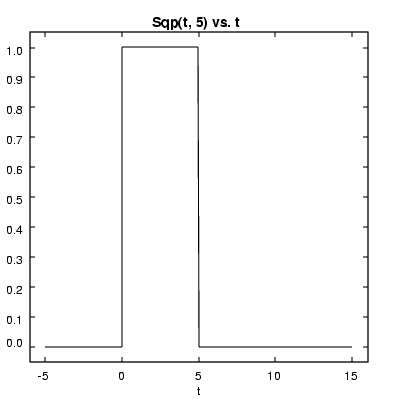sqw(t, d, p) square wave of period p with duty cycle d% at time t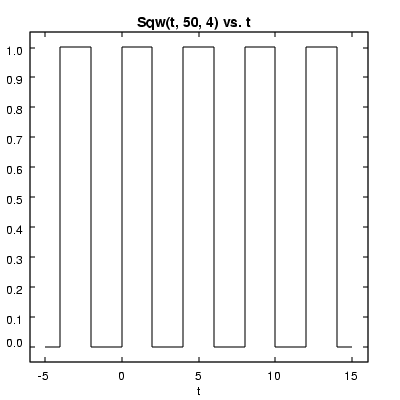stair(t, l) stair function at time t with length l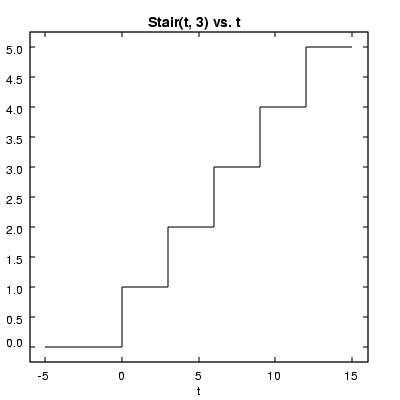step(t) step function at time t (0 if t < 0, 1 otherwise)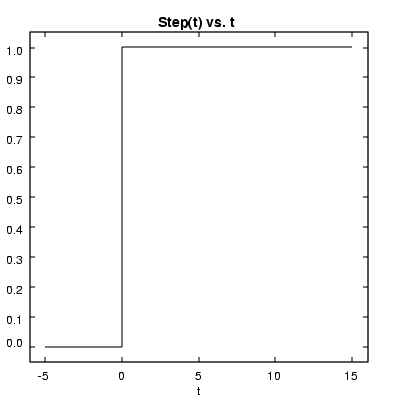swp(t, l) sawtooth pulse at time t with length l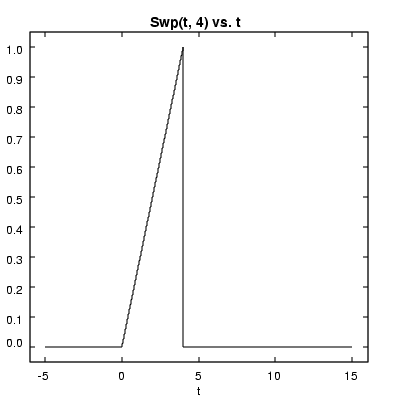sww(t, d, p) sawtooth wave with duty cycle d% and period p at time t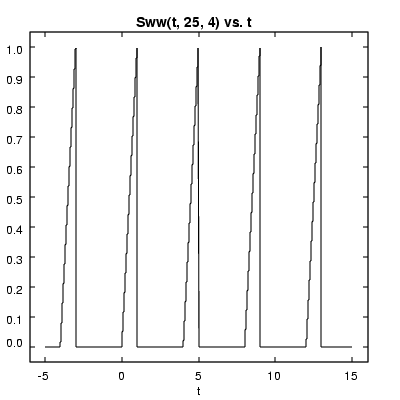trp(t, l) triangle wave pulse at time t with length l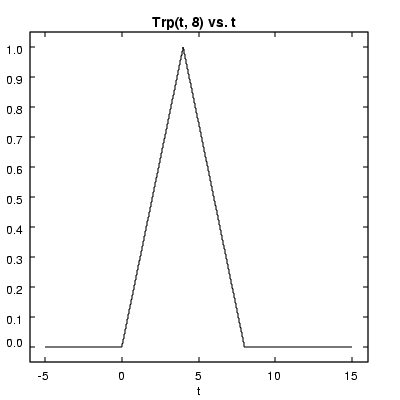trw(t, d, p) triangle wave at time t with duty cycle d% and period p